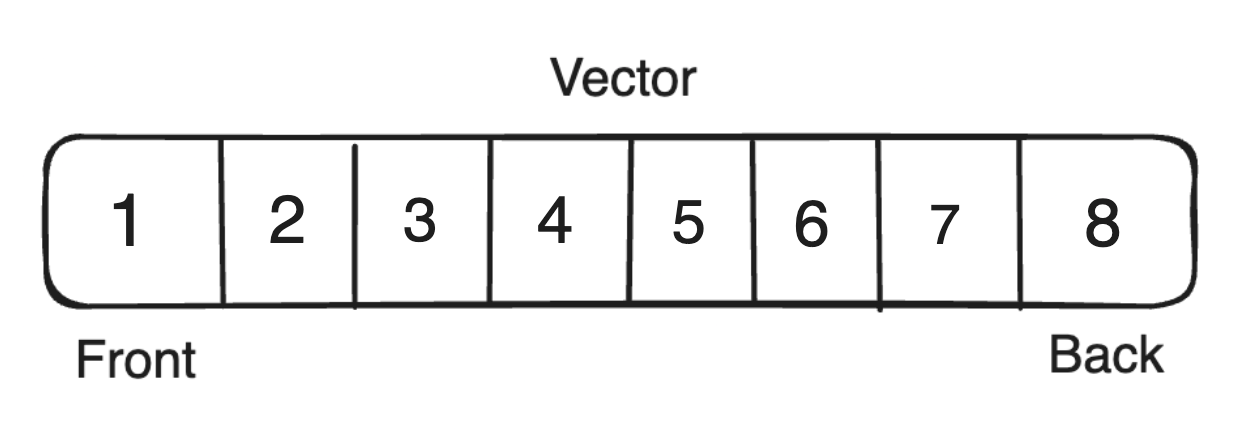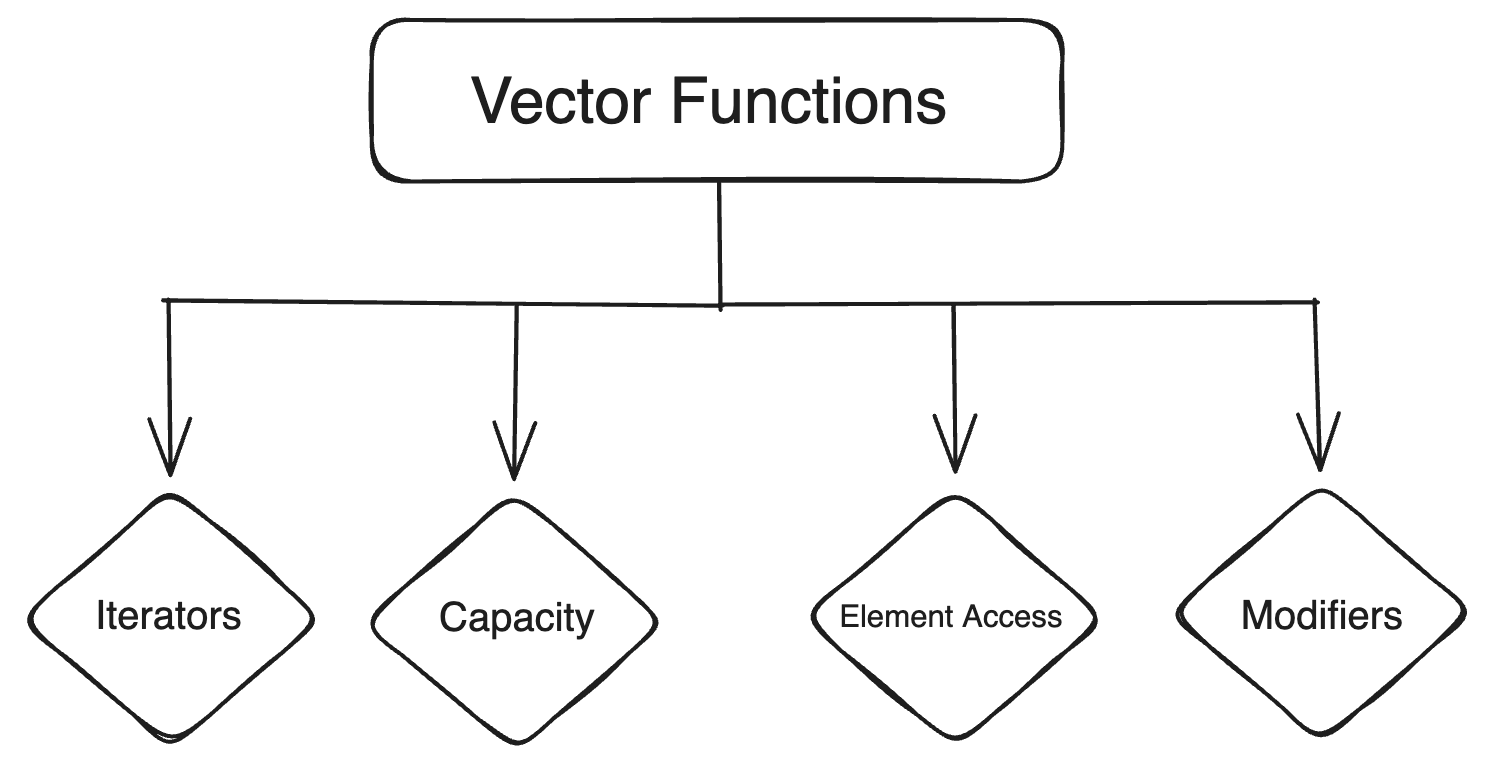# Vectors in C++ & Vector Functions (with Examples)

Vectors are an essential data structure in C++ that provide a dynamic array-like structure, allowing for the efficient storage and manipulation of elements. Whether you're a beginner or an experienced programmer, understanding vectors in C++ is crucial for building robust and flexible applications. This comprehensive guide will explore the concept of vectors, their functionalities, and how to effectively utilize them in your programs.

## What are Vectors in C++?

Vectors in C++ are sequential containers that store elements in a continuous order. They can dynamically change their size at runtime, automatically adjusting to accommodate new elements. Unlike arrays, which have a fixed size, vectors provide flexibility and convenience in managing collections of data. Vectors can store elements of any data type, such as integers, characters, or even user-defined objects.## Declaration of Vectors in C++

To use vectors in C++, you need to include the header file. The syntax for declaring a vector is as follows:

```std::vector<type> vector_name;
```

Here, type represents the data type of the elements that will be stored in the vector, and vector_name is the neme given to the vector. For example, to declare a vector of integers named numbers, you can use the following code:

```std::vector<int> numbers;
```

Unlike arrays, you don't need to specify the size of the vector during declaration, as vectors can dynamically resize themselves as elements are added or removed.

## Initialization of Vectors in C++

There are several ways to initialize a vector in C++. Let's explore some common initialization methods.

### Method 1: Initializer List

You can initialize a vector by providing a list of values enclosed in curly braces. For example:

```std::vector<int> numbers = {1, 2, 3, 4, 5};
```

The vector numbers are initialized with the values 1, 2, 3, 4, and 5.

### Method 2: Uniform Initialization

C++11 introduced uniform initialization syntax, which allows you to initialize a vector without using the equals sign. For example:

```std::vector<int> numbers {1, 2, 3, 4, 5};
```

This syntax is equivalent to the previous method and initializers the vector numbers with the same values.

### Method 3: Using the std::vector Constructor

You can also initialize a vector by using the constructor of the std::vector class. The constructor method allows you to specify the size of the vector and the initial value for all elements. For example:

```std::vector<int> numbers(5, 0);
```

Here, the vector numbers are initialized with a size of 5 and all elements set to 0.

### Method 4: Using an Existing Vector

If you have an existing vector, you can create a new vector with the same values using the constructor. For example:

```std::vector<int> numbers1 = {1, 2, 3, 4, 5};
std::vector<int> numbers2(numbers1.begin(), numbers1.end());
```

Here, the vector numbers2 is initialized with the values of numbers. The begin() and end() functions are used to retrieve the iterators pointing to the first and last elements of numbers1, respectively.

You can read this article to explore more methods: Initialize a vector in C++ ( 8 Easy Methods )

## Various Functions in Vectors

Vectors in C++ provide a wide range of member functions that enables you to perform various operations on the vector. Let's explore some commonly used functions.### 1. Iterators

Iterators are used to traverse or iterate over the elements of a vector. They provide a way to access and manipulate the elements in a sequential manner. Here ere seme commonly used iterator functions:

• begin(): Returns an iterator pointing to the first element of the vector.
• end(): Returns an iterator pointing to the element following the last element ef the vector.
• cbegin(): Returns a constant iterator pointing to the first element of the vector.
• cend(): Returns a constant iterator pointing to the element following the last element of the vector.
• rbegin(): Returns a reverse iterator pointing to the last element of the vector.
• rend(): Returns a reverse iterator pointing to the element preceding the first element ef the vector.
• crbegin(): Returns a constant reverse iterator pointing to the last element of the vector.
• crend(): Returns a constant reverse iterator pointing to the element preceding the first element ef the vector.

These iterator functions allow you to iterate over the elements of a vector using a loop or perform other operations on the elements.

Example:

```std::vector<int> numbers = {1, 2, 3, 4, 5};

// Using iterators to traverse the vector
std::cout << "Output of begin and end: ";
for (auto it = numbers.begin(); it != numbers.end(); ++it) {
std::cout << *it << " ";
}

// Using const iterators to traverse the vector
std::cout << "\nOutput of cbegin and cend: ";
for (auto it = numbers.cbegin(); it != numbers.cend(); ++it) {
std::cout << *it << " ";
}

// Using reverse iterators to traverse the vector
std::cout << "\nOutput of rbegin and rend: ";
for (auto rit = numbers.rbegin(); rit != numbers.rend(); ++rit) {
std::cout << *rit << " ";
}

// Using const reverse iterators to traverse the vector
std::cout << "\nOutput of crbegin and crend: ";
for (auto rit = numbers.crbegin(); rit != numbers.crend(); ++rit) {
std::cout << *rit << " ";
}
```

Output:

```Output of begin and end: 1 2 3 4 5

Output of cbegin and cend: 1 2 3 4 5

Output of rbegin and rend: 5 4 3 2 1

Output of crbegin and crend: 5 4 3 2 1
```

In the above example, we use different iterator functions to traverse vector numbers and print its elements.

### 2. Capacity

Capacity functions provide information about the size and storage capacity of a vector. Here ere seme commonly used capacity functions:

• size(): Returns the number of elements in the vector.
• max_size(): Returns the maximum number of elements that the vector can hold.
• empty(): Returns a boolean value indicating whether the vector is empty or not.
• resize(n): Resize the vector so that it contains n elements.
• capacity(): Returns the current storage capacity of the vector.
• reserve(n): Requests that the vector capacity be at least enough to contain n elements.

These capacity functions allow you to manage the seze and storage of the vector efficiently.

Example:

```std::vector<int> numbers = {1, 2, 3, 4, 5};

std::cout << "Size: "  << numbers.size() << std::endl;
std::cout << "Max Size: " << numbers.max_size() << std::endl;
std::cout << "Empty: " << (numbers.empty() ? "Yes" : "No") << std::endl;

numbers.resize(10);
std::cout << "After Resize: Size = " << numbers.size() << ", Capacity = " << numbers.capacity() << std::endl;

numbers.reserve(20);
std::cout << "After Reserve: Size = " << numbers.size() << ", Capacity = " << numbers.capacity() << std::endl;
```

Output:

```Size: 5
Max Size: 4611686018427387903
Empty: No
After Resize: Size = 10, Capacity = 10
After Reserve: Size = 10, Capacity = 20
```

In the above example, we use various capacity functions to obtain information about the size and capacity of the vector numbers. We also demonstrate how to resize and reserve capacity for the vector.

### 3. Element Access

Element access functions allow you to access and manipulate individual elements of a vector. Here ere seme commonly used element access functions:

• operator[]: Returns a reference to the element at the specified index.
• at(): Returns a reference to the element at the specified index, with bounds checking.
• front(): Returns a reference to the first element ef the vector.
• back(): Returns a reference to the last element ef the vector.
• data(): Returns a pointer to the underlying array that stores the elements.

These element access functions provide convenient ways to retrieve and modify specific elements in a vector.

Example:

```std::vector<int> numbers = {1, 2, 3, 4, 5};

std::cout << "Element at Index 0: " << numbers << std::endl;
std::cout << "Element at Index 2: " << numbers << std::endl;
std.::cout << "Element at Index 4: " << numbers << std::endl;

std::cout << "Front Element: " << numbers.front() << std::endl;
std::cout << "Back Element: " << numbers.back() << std::endl;

int* ptr = numbers.data();
std::cout << "Data Pointer: " << *ptr << std::endl;
```

Output:

```Element at Index 0: 1
Element at Index 2: 3
Element at Index 4: 5
Front Element: 1
Back Element: 5
Data Pointer: 1
```

In the above example, we use different element access functions to retrieve specific elements of the vector numbers and demonstrate how to access the underlying array using the data() function.

### 4. Modifiers

Modifiers are functions that allow you to add, remove, or modify elements in a vector. Here ere seme commonly used modifier functions:

• push_back(): Adds an element to the end ef the vector.
• pop_back(): Removes the last element from the vector.
• insert(): Inserts an element at the specified position.
• erase(): Removes elements from the vector at the specified position or range.
• swap(): Swaps the contents of two vectors.
• assign(): Assigns new values to the vector, replacing the old values.
• clear(): Removes all elements from the vector.
• emplace(): Inserts a new element at the specified position in the vector.
• emplace_back(): Adds a new element to the end ef the vector.

These modifier functions provide flexibility in managing the elements of a vector.

Example:

```std::vector<int> numbers;

numbers.push_back(1);
numbers.push_back(2);
numbers.push_back(3);

numbers.pop_back();

numbers.insert(numbers.begin() + 1, 5);
numbers.insert(numbers.end(), 6);

numbers.erase(numbers.begin() + 2);

std::vector<int> otherNumbers = {10, 20, 30};
numbers.swap(otherNumbers);

numbers.assign(3, 100);

numbers.clear();
```

In the above example, we demonstrate the use of various modifier functions to add, remove, and modify elements in the vector numbers. We also show how to swap the contents of two vectors, assign new values to a vector, and clear a vector.

## Conclusion

In this guide, we explored the concept of vectors in C++ and delve into their functionalities and usage. Vectors provide a flexible and efficient way to store and manipulate collections of elements. By understanding the various member functions of vectors, you can confidently utilize vectors in your C++ programming projects.

### FavTutor - 24x7 Live Coding Help from Expert Tutors!